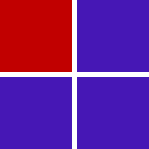# Fraction to Decimal Converter

Simple Fraction
Mixed Fraction
Enter fraction:
Decimals to Round to:
Convert
Swap
Reset
Decimal result:
11
/
16
= 0.6875
Calculation:
0
11
/
16
=
0
+
11
/
16
We know that:
11
/
16
is the same as
11 ÷ 16
Therefore:
0
11
/
16
=
0 + ( 11 ÷ 16 )
Then using Long Division for
11 divided by 16
and rounding to a Max of 'Do Not Round' Decimal Places
we have
= ( 0 + 0.6875 ) = 0.6875

## What is Fraction?

What is a fraction, also called parts of a while, so we could call it parts of a whole, we could call it what is a fraction.

Lots of students have problems with fractions and it actually fractions is one of the most important things you will ever learn in math and we can take and break it apart and make it understandable because the little secret is fractions are actually really easy to understand.

Basically let's go back and reverse a little bit for a second, we all know how to count numbers. Let's say we have three oranges, there's orange #1, orange #2, orange #3, we know how to count objects, fractions let us talk about when we have less than one of an object, so what we need to do is understand what fractions are and also how to write them down so that we understand exactly what they are.

So let's say we have a square, and we can consider this square as a whole. Now, divide this square into 4 equal parts, now select one of those equal parts (check pic below).So the question is what fraction of the whole is the part that has shaded in red? Well, it is 1 out of the 4 equal parts. We have shaded in 1 out of 1, 2, 3, 4 equal parts. So we write this as fraction this piece represents 1/4 of the whole. You could view this as 1 of the 4 equal parts. But remember, it needs to be 4 equal parts.

## How to convert fraction to decimal

We are going to cover conversion of fraction to decimal.

Example #1

Convert 7/16 to decimal:

We know that:
7/16 = 7 ÷ 16
Then using Long Division for 7 divided by 16
7÷16 = 0.4375

Example #2

Convert 1/4 to decimal:

 0.25 4 1 0 10 8 20 20 0

Use long division to divide the fraction's numerator by the fraction's denominator. The fraction numerator will be the dividend and the denominator will be the divisor. Rearrange the fraction into long division form. Add a decimal and trailing zeros. You're done! That's it, the fraction is now in decimal form.

Example #3

Convert mixed fraction 2 5/8 to decimal:

2 5/8 = 2+5/8
We know that: 5/8 is the same as 5 ÷ 8
Therefore:
2 5/8 = 2 + (5 ÷ 8)
Then using Long Division for 5 divided by 8 we have
2 + 0.625 = 2.625

Example #4

Convert 3/5 to decimal:

3/5 = 3*2/5*2 = 6/10 = 0.6

3/5 is expanded to 6/10 by multiplying the numerator by 2 and denominator by 2.

FractionDecimal
1/20.5
1/30.333333
1/40.25
1/50.2
1/60.166667
1/70.142857
1/80.125
1/90.111111
1/100.1
2/50.4
3/50.6
4/60.666667
2/70.285714
3/70.428571
4/70.571429
5/60.833333
3/80.375
8/90.888889
3/190.157895
5/220.227273
8/130.615385
9/100.9
10/1000.1
100/1010.990099
1000/99990.10001